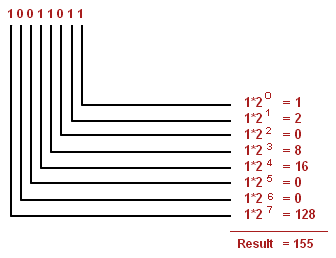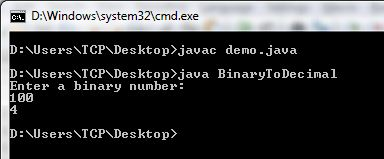• /*二进制转化为十进制*/ int nums;//输入的二进制的数 int numss = 0;//输出的十进制的数 int i; int n;//输入几位数 int x = {1,2,4,8,16.32,64,128,256,512,1024}; ...
#include<stdio.h>
int main()
{
/*二进制转化为十进制*/
int nums;//输入的二进制的数

int numss = 0;//输出的十进制的数

int i;

int n;//输入几位数
int x = {1,2,4,8,16.32,64,128,256,512,1024};
printf("请输入二进制的位数；");
scanf("%d",&n);
printf("请输入；");
for(i = 0; i < n;i ++)
{
scanf("%d",&nums[i]);
}

for(i = 0; i < n ;i ++)
{
numss = numss + (nums[i] * x[n - i - 1]);
}

printf("十进制：%d\n",numss);
return 0;

}




展开全文• 十进制转化为二进制： code： #include #include #include using namespace std; int main() { int num=10; char str; itoa(num,str,2); printf("%08s\n",str);...将二进制转化为十进制。 code： #inc
十进制转化为二进制：
code：
#include <iostream>
#include <cstdlib>
#include <cstdio>
using namespace std;

int main() {
int num=10;
char str;
itoa(num,str,2);
printf("%08s\n",str);
return 0;
}
将二进制转化为十进制。
code：
#include<cstdio>
int main()
{
int num = 10;
char str;
int n = atoi(itoa(num, str, 2));
printf("%d\n",n);
return 0;
}
先把num转换为二进制的字符串，再把该字符串转换为整数。
展开全文• java二进制转化为十进制Here you will get program to convert binary to decimal in Java. 在这里，您将获得在Java中将二进制转换为十进制的程序。 There are mainly two ways to convert a binary number to ...java二进制转化为十进制Here you will get program to convert binary to decimal in Java.
在这里，您将获得在Java中将二进制转换为十进制的程序。
There are mainly two ways to convert a binary number to decimal number in Java.
在Java中，主要有两种将二进制数转换为十进制数的方法。
1. By using parseInt() method of Integer class.
2. By using user defined logic.
1.通过使用Integer类的parseInt()方法。 2.通过使用用户定义的逻辑。
用Java将二进制转换为十进制的程序 (Program to Convert Binary to Decimal in Java)
By using Integer.parseInt()
通过使用Integer.parseInt()
Integer.parseInt() method takes two arguments. First argument is a string and second argument is the base or radix in which we have to convert the number. The output is the integer represented by the string argument in the specified radix. Below is the program for it.
Integer.parseInt()方法采用两个参数。 第一个参数是一个字符串，第二个参数是我们必须在其中转换数字的基数或基数。 输出是由指定基数中的字符串参数表示的整数。 下面是它的程序。
import java.util.Scanner;

class BinaryToDecimal
{
public static void main(String args[])
{
Scanner s=new Scanner(System.in);

System.out.println("Enter a binary number:");

String n=s.nextLine();

System.out.println(Integer.parseInt(n,2));
}
}
Without using Integer.parseInt()
不使用Integer.parseInt()
In this method we have to define our own logic for converting binary number to decimal. The approach that we will use here is mentioned in below example.
在这种方法中，我们必须定义自己的逻辑，以将二进制数转换为十进制。 下面的示例中提到了我们将在此处使用的方法。Image Source
图片来源
The program that implements above approach is mentioned below.
下面介绍实现上述方法的程序。
import java.util.Scanner;

class BinaryToDecimal
{
public static void main(String args[])
{
Scanner s=new Scanner(System.in);

System.out.println("Enter a binary number:");
int n=s.nextInt();

int decimal=0,p=0;

while(n!=0)
{
decimal+=((n%10)*Math.pow(2,p));
n=n/10;
p++;
}

System.out.println(decimal);
}
}
Output
输出量If you found anything missing or incorrect in above programs then please mention it by commenting below.
如果您在上述程序中发现任何丢失或不正确的内容，请在下面的评论中提及。
翻译自: https://www.thecrazyprogrammer.com/2015/11/program-to-convert-binary-to-decimal-in-java.htmljava二进制转化为十进制
展开全文java python 编程语言 spring
• 我们以 -1 为例十进制 1 的二进制是 0000 0001 大致算法如下：1x2^0+0x2^1+0x2^2+0x2^3+0x2^4+0x2^5+0x2^6+0x2^7 =1那么 -1 怎么转化为二进制呢？负数的二进制是以负数的绝对值的补码来进行计算的，比如：1 的二进制...
大家都知道我们平时生活中用的都是十进制，但是计算机使用二进制，那么二进制与十进制如何进行相互转化。下面我们来看一看。我们以 -1 为例十进制 1 的二进制是 0000 0001 大致算法如下：1x2^0+0x2^1+0x2^2+0x2^3+0x2^4+0x2^5+0x2^6+0x2^7 =1那么 -1 怎么转化为二进制呢？负数的二进制是以负数的绝对值的补码来进行计算的，比如：1 的二进制是 0000 0001 反码是 1111 1110补码是反码加 1补码就是 1111 1111所以 -1 的二进制就是 1111 1111当然十进制转化为二进制是相同的原理。
展开全文• c语言二进制转化为十进制Here you will get program to convert decimal to binary in C. 在这里，您将获得将C中的十进制转换为二进制的程序。 We can convert a decimal number into binary by repeatedly ...python 算法 c++ c语言 java
• 二进制转化为十进制 ①按权展开方法Java实现 /* * 按权展开法 */ public static double BinToTen1(String binary) { //查找改二进制是否存在小数点 int index = binary.indexOf('.'); //转化成的十进制 ...
• 二进制转化为十进制（除10取余法） 除10取余法中，对于二进制转化为10进制来讲要除于1010，就是把10转化为二进制数，再用二进制数除于1010 例如：二进制11101除于1010，余数1001，转化为十进制数为9，这个是相当于十...
• 二进制转化为十进制数 用字符型接收二进制的0、1然后判断是否为‘1’ 功能实现 代码片. #include<iostream> using namespace std; double powe(double x, int n); int main() { int su = 0; char a; for ...c++
• c语言编程-二进制转化为十进制 #include<stdio.h> static int a=0; double bintodec(char*str) { a=a*2+(*str-'0'); return 0; } int main() { char ch; while(ch=getchar(),ch!='\n') { char *p=&...
• 二进制转化为十进制①按权展开方法Java实现/** 按权展开法*/public static double BinToTen1(String binary) {//查找该二进制是否存在小数点int index = binary.indexOf('.');//转化成的十进制double ten = 0;//整数...
• 利用栈的特性将二进制转化为十进制 10001000 -> 136 如上公式，可将二进制入栈。然后一次出栈，将其值进行处理，求和。即得到十进制值。 下边是自己实现的栈以及将二进制转化为十进制的代码 public class ...java实现栈
• # 关于二进制转化为十进制的问题 ` #include #include using namespace std; int main() { int n; //二进制数 int count; //用来存储数字个数 cin >> count ; cin >> n; int * p = new int...c++
• 二进制转化为十进制的深程刨析： （位运算符） public int getDecimalValue(ListNode head) { ListNode cur = head; int ans = 0; while (cur != null) { ans <<= 1; ans += cur.val; cur = cur.next; ...
• 根据十进制转换二进制的思想：把这个数除以2若单数则1，偶数则0，直到最后一个数1为止。所以我们要做的就是用你给定的这个数除以2，如果结果奇数则r=1,否则r=0;如此循环下去，直到这个数...
• ”通过之前的学习，我们已经知道了二进制是计算机的语言，可以说没有二进制就没有现在的计算机，那如何把二进制转化为我们熟悉的十进制呢？点击边框调出视频工具条天天我们认识数的时候，都是从数数开始的，让我们先...
• 1.python二进制转化十进制 s = input() # 请输入一个由1和0组成的二进制数字串： d = 0 while s: d = d*2 + (ord(s) -ord('0')) s = s[1:] print(&quot;转换成八进制数是：{:}&quot;.format(d)...
• 2. IP地址二进制范围：00000000.00000000.00000000.00000000-11111111.11111111.11111111.11111111IP地址十进制范围：0.0.0.0-255.255.255.2553. IP地址二进制排位顺序是从右到左。顺序依次...
• 我们说说这个二进制十进制之间的关系与转化。 我们非常熟悉十进制的表现形式，也就是大家平时所用的1,2,3，....，这叫数制的一种表现形式，我们称为十进制。但是这并不是唯一的数制表现形式，比如说，大家平时所说...
• 这个学期迫于学习数据结构，没办法，把书上的例子重新敲了一遍。 使用栈实现二进制转化为十进制
• 比如二进制1101，知换算成十进制就是：1*2（1-1）+0*2（2-1）+1*2（3-1）+1*2（4-1）=1+0+4+8=13。 扩展资道料： 1、二进制转换八进制： 把二进制的数从右往左，三位一组，不够补0 列：111=4+2+1=7 11001拆
• 二进制转化为十进制，是从一串二进制数的末尾（右边）开始，每位上数（0或1）×2^该数是第几个（从0开始）。 例如：二进制数1101.01转化成十进制 1101.01（2）=1*20+0*21+1*22+1*23 +0*2-1+1*2-2=1+0+4+8+0+0.25=...数据结构 C语言...